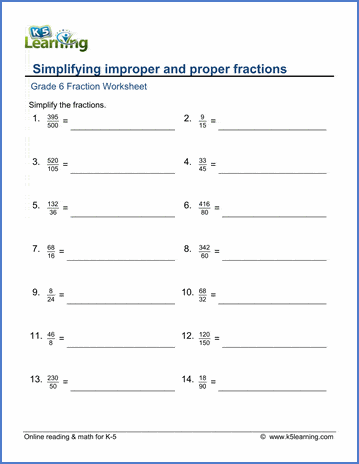# Simplifying Fractions for Grade 6

## Equivalent fractions math worksheets

These grade 6 math worksheets provide practice in simplifying fractions, recognizing equivalent fractions and converting fractions to and from mixed numbers.

## Fractions to/from mixed numbers

Convert mixed numbers to fractions 15 12/15 =
Fractions to mixed numbers 9/5 =
Fractions to mixed numbers (harder) 155/16 =

## Simplify fractions

Simplify fractions 15/50 =
Simplify fractions (harder) 33/111 =
Simplify improper fractions 50/35 =

## Equivalent fractions

Equivalent fractions (2 fractions) 3/4 = 6 /__
Equivalent fractions (3 fractions) 3/4 = 6/__=9/__
Equivalent fractions (4 fractions) 3/4=6/__=9/__= __/20Sample Grade 6 Simplifying Fractions Worksheet

## More fractions worksheets

Explore all of our fractions worksheets, from dividing shapes into "equal parts" to multiplying and dividing improper fractions and mixed numbers.

What is K5?

K5 Learning offers free worksheets, flashcards and inexpensive workbooks for kids in kindergarten to grade 5. Become a member to access additional content and skip ads.